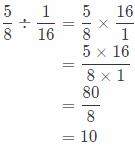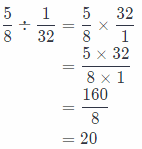# Texas Go Math Grade 6 Lesson 3.3 Answer Key Multiplying Dividing Fractions

Refer to our Texas Go Math Grade 6 Answer Key Pdf to score good marks in the exams. Test yourself by practicing the problems from Texas Go Math Grade 6 Lesson 3.3 Answer Key Multiplying Dividing Fractions.

## Texas Go Math Grade 6 Lesson 3.3 Answer Key Dividing Fractions

Reflect

Question 1.
Write the division shown by each model.
In A, the total amount is given by the fraction $$\frac{3}{4}$$ and the divisor is $$\frac{1}{8}$$, therefore the division expression is $$\frac{3}{4} \div \frac{1}{8}$$

In B, the total amount is given by the fraction $$\frac{1}{2}$$ and the divisor is 5, therefore the division expression is $$\frac{1}{2}$$ ÷ 5.

Is any number its own reciprocal? If so, what number(s)? Justify your answer.
OnLy 1 and – 1 are number which are its own reciprocal.
We can write 1 as a fraction on following way;
1 = $$\frac{1}{1}$$
The reciprocal we can find switching numerator and denominator and get:
$$\frac{1}{1}$$ = 1
So, conclusion is that 1 is its own reciprocal.
Also, – 1 we can write as a fraction on the following way:
– 1 = $$\frac{-1}{1}$$
We can find the reciprocal switching numerator and denominator and get:
$$\frac{1}{-1}$$ = – 1
So, Conclusion is that – 1 is its own reciprocal.

Question 3.
Communicate Mathematical Ideas Does every number have a reciprocal? Explain.
Any number except 0 has a reciprocal. 0 has no reciprocal because of the following:
0 = $$\frac{0}{1}$$
But if switch the numerator and denominator, we will get $$\frac{1}{0}$$ but fraction is not defined when denominator is 0.
Because of previous, 0 is the only number which has no reciprocal.

Question 4.
The reciprocal of a whole number is a fraction with ___________ in the numerator.
Missing word is 1. For example:
6 = $$\frac{6}{1}$$
Its reciprocal is $$\frac{1}{6}$$.
So, always, the reciprocal of the whole number is a fraction with 1 in the numerator.
Missing word (number) is 1.

Find the reciprocal of each number.

Question 5.
$$\frac{7}{8}$$
We have to switch the numerator and the denominator in order to find the reciprocal. After switching, we get that the reciprocal is $$\frac{8}{7}$$.

Question 6.
9
First, we will rewrite 6 as a fraction:
6 = $$\frac{6}{1}$$
Now, we will switch the numerator and the denominator and get that reciprocal of 6 is:
$$\frac{1}{6}$$

Question 7.
$$\frac{1}{11}$$
We have to switch the numerator and the denominator in order to find the reciprocal. After switching, we get that the reciprocal is $$\frac{11}{1}$$ = 11.

Reflect

Question 8.
Make a Conjecture Use the pattern in the table to make a conjecture about how you can use multiplication to divide one fraction by another.
From the table we can see that division is actually multiplying the one fraction by reciprocal of the other fraction
So, first we have to find reciprocal of the other fraction and by it multiplying the first one.

Division is multiplying one fraction by reciprocal of the other fraction.

Write a division problem and a corresponding multiplication problem like those in the table. Assuming your conjecture in 8 is correct, what is the answer to your division problem?
For example, we would divide the following fractions:
$$\frac{6}{7} \div \frac{3}{4}=\frac{8}{7}$$
Now, we will divide those two fractions as we explained at previous task. Reciprocal of $$\frac{3}{4}$$ is $$\frac{4}{3}$$
Next thing we will do will be to multiply $$\frac{6}{7}$$ by $$\frac{4}{3}$$.We can see that those two results are equals, so, this explained way for dividing to fractions is correct.
$$\frac{6}{7} \div \frac{3}{4}=\frac{6}{7} \times \frac{4}{3}=\frac{8}{7}$$

Divide.

Question 10.
$$\frac{9}{10} \div \frac{2}{5}$$
First, we have to find reciprocal of $$\frac{3}{5}$$, it is $$\frac{5}{3}$$. Then multiply $$\frac{9}{10}$$ by $$\frac{5}{2}$$ multiplying their numerators and denominators and then write result in the simplest form:So, $$\frac{9}{10} \div \frac{2}{5}=\frac{9}{4}$$

Question 11.
$$\frac{9}{10} \div \frac{3}{5}$$
First, we have to find reciprocal of $$\frac{3}{5}$$, it is $$\frac{5}{3}$$. Then multiply $$\frac{9}{10}$$ by $$\frac{5}{3}$$ multiplying their numerators and denominators and then write result in the simplest form: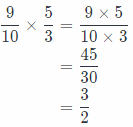So, $$\frac{9}{10} \div \frac{3}{5}$$ = $$\frac{3}{2}$$

Find the reciprocal of each fraction.

Question 1.
$$\frac{2}{5}$$
In order to find the reciprocal of $$\frac{2}{5}$$, we have to switch the numerator and denominator. So, applying this we get that reciprocal of $$\frac{2}{5}$$ is $$\frac{5}{2}$$.

Question 2.
$$\frac{1}{9}$$
In order to find the reciprocal of $$\frac{1}{9}$$, we have to switch the numerator and denominator. So, applying this we get that reciprocal of $$\frac{1}{9}$$ is $$\frac{9}{1}$$ = 9.

Lesson 3.3 Dividing Fractions Answer Key Question 3.
$$\frac{10}{3}$$
In order to find the reciprocal of $$\frac{10}{3}$$, we have to switch the numerator and denominator. So, applying this we get that reciprocal of $$\frac{10}{3}$$ is $$\frac{3}{10}$$

Divide.

Question 4.
$$\frac{4}{3} \div \frac{5}{3}$$
First, we have to find the reciprocal of $$\frac{5}{3}$$, it is $$\frac{3}{5}$$. Then multiply $$\frac{4}{3}$$ by $$\frac{3}{5}$$, multiplying their nominators and denominators and then write the result in the simplest form: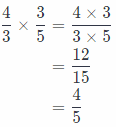So, $$\frac{4}{3} \div \frac{5}{3}$$ = $$\frac{4}{5}$$

Question 5.
$$\frac{3}{10} \div \frac{4}{5}$$
First, we have to find the reciprocal of $$\frac{4}{5}$$, it is $$\frac{5}{4}$$. Then multiply $$\frac{3}{10}$$ by $$\frac{5}{4}$$, multiplying their nominators and denominators and then write the result in the simplest form: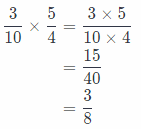So, $$\frac{3}{10} \div \frac{4}{5}$$ = $$\frac{3}{8}$$

Question 6.
$$\frac{1}{2} \div \frac{2}{5}$$
First, we have to find the reciprocal of $$\frac{2}{5}$$, it is $$\frac{5}{2}$$. Then multiply $$\frac{1}{2}$$ by $$\frac{5}{2}$$, multiplying their nominators and denominators and then write the result in the simplest form:So, $$\frac{1}{2} \div \frac{2}{5}$$ = $$\frac{5}{4}$$

Essential Question Check-In

Question 7.
How do you divide fractions?
We divide two fractions on that way we actually multiply the first one by reciprocal of the second fraction.

Question 8.
Alison has $$\frac{1}{2}$$ cup of yogurt for making fruit parfaits. Each parfait requires $$\frac{1}{8}$$ cup of yogurt. How many parfaits can she make?
In order to find how many parfaits Alison can make, we have to divide $$\frac{1}{2}$$ by $$\frac{1}{8}$$ and get: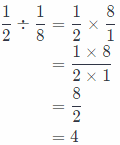So, she can make 4 par falts with $$\frac{1}{2}$$ cup of yogurt.

Question 9.
A team of runners is needed to run a $$\frac{1}{4}$$-mile relay race. If each runner must run $$\frac{1}{16}$$ mile, how many runners will be needed?
In order to find how many parfaits Alison can make, we have to divide $$\frac{1}{4}$$ by $$\frac{1}{16}$$ and get:So, there will be needed 4 runners.

Trevor paints $$\frac{1}{6}$$ of the fence surrounding his farm each day. How many days will it take him to paint $$\frac{3}{4}$$ of the fence?
If we want to find how many days will take Trevor to point $$\frac{3}{4}$$ of the fence, we need to divide $$\frac{3}{4}$$ by $$\frac{1}{6}$$ and get: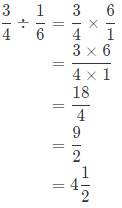So, Trevor will take 4 and a half days to paint $$\frac{3}{4}$$ of the fence.

Question 11.
Six people share $$\frac{3}{5}$$ pound of peanuts equally. What fraction of a pound of peanuts does each person receive?
In order to calculate what fraction of a pound of peanuts each person receives, we have to divide $$\frac{3}{5}$$ by 6 and get:So, each person receives $$\frac{1}{10}$$ of pound of peanuts.

Question 12.
Biology If one honeybee makes $$\frac{1}{12}$$ teaspoon of honey during its lifetime, how many honeybees are needed to make $$\frac{1}{2}$$ teaspoon of honey?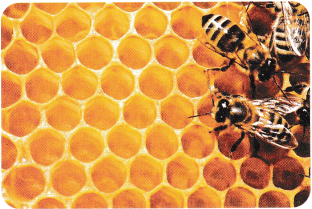In order to calculate how many noneybees are needed to make $$\frac{1}{2}$$ teaspoon of honey, we have to divide $$\frac{1}{2}$$ by $$\frac{1}{12}$$ and get:So, 6 honeybees are needed to make $$\frac{1}{2}$$ teaspoon of honey

Question 13.
Jackson wants to divide a $$\frac{3}{4}$$ -pound box of trail mix into small bags. Each of the bags will hold $$\frac{1}{12}$$ pound of trail mix. How many bags of trail mix can Jackson fill?
If we want to find how many bags of trail mix Jackson can fill, we have to divide $$\frac{3}{4}$$ by $$\frac{1}{12}$$ and get: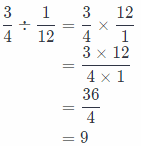So, Jackson can fill 9 bags of trail mix.

Question 14.
A pitcher contains $$\frac{2}{3}$$ quart of lemonade. If an equal amount of lemonade is poured into each of 6 glasses, how much lemonade will each glass contain?
In order to find how much Lemonade each glass will contain, we have to divide $$\frac{2}{3}$$ by 6 and get: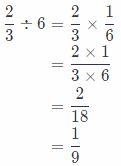So, each glass will contain $$\frac{1}{9}$$ of lemonade.

Question 15.
How many tenths are there in $$\frac{4}{5}$$?
To calculate how many tenths are in $$\frac{4}{5}$$, we need to divide $$\frac{4}{5}$$ by 10 and get: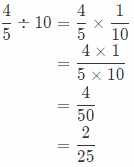So, there are $$\frac{2}{25}$$ tenths in $$\frac{4}{5}$$.

Lesson 3.3 Multiplying and Dividing Fractions Question 16.
You make a large bowl of salad to share with your friends. Your brother eats $$\frac{1}{3}$$ of it before they come over.

a. You want to divide the leftover salad evenly among six friends. What expression describes the situation? Explain.
If there was $$\frac{3}{3}$$ = 1 salad and brother eats $$\frac{1}{3}$$ of it, we suppose to subtract $$\frac{1}{3}$$ from $$\frac{3}{3}$$ and the result will be the leftover.
Then, that leftover we need to divide among six friends, so $$\frac{3}{5}$$ – $$\frac{1}{3}$$ we need to divide by 6.
The following expression describes the situation in the task:
$$\left(\frac{3}{3}-\frac{1}{3}\right)$$ ÷ $$\frac{1}{6}$$

b. What fractional portion of the original bowl of salad does each friend receive?
Required fractional portion of the original bowl of salad each friends receives is result of following expression:So, each friend receive $$\frac{1}{6}$$ of the original salad.

H.O.T. Focus On Higher Order Thinking

Question 17.
Interpret the Answer The length of a ribbon is $$\frac{3}{4}$$ meter. Sun Yi needs pieces measuring $$\frac{1}{3}$$ meter for an art project. What is the greatest number of pieces measuring $$\frac{1}{3}$$ meter that can be cut from the ribbon? How much ribbon will be left after Sun Yi cuts the ribbon? Explain your reasoning.
The greatest number of pieces measuring $$\frac{1}{3}$$ meter that can be cut from the ribbon is: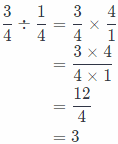So, there are 3 pieces whose length is $$\frac{1}{3}$$ meter and can be cut from the ribbon whose length $$\frac{1}{3}$$ meter
So, if Sun Yi cuts one piece of length $$\frac{1}{3}$$ meter, there will be Left 2 more pieces whose length is $$\frac{1}{3}$$ or
$$2 \times \frac{1}{3}=\frac{2 \times 1}{1 \times 3}=\frac{2}{3}$$ = meter
So, there will be left $$\frac{2}{3}$$ meter of ribbon.

Question 18.
Represent Real-World Problems Liam has $$\frac{9}{10}$$ gallon of paint for painting the birdhouses he sells at the craft fair. Each birdhouse requires $$\frac{1}{20}$$ gallon of paint. How many birdhouses can Liam paint? Show your work.
In order to find how many birdhouses Liam can paint, we need to divide $$\frac{9}{10}$$ by $$\frac{1}{20}$$ and get: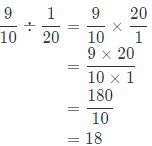Liam can paint 18 birdhouses.

Question 19.
Justify Reasoning When Kaitlin divided a fraction by $$\frac{1}{2}$$, the result was a mixed number. Was the original fraction less than or greater than $$\frac{1}{2}$$? Explain your reasoning.
Conclusion is that the original fraction was greater than $$\frac{1}{2}$$.
Realty, for example let the original fraction be $$\frac{4}{3}$$, it is greater than $$\frac{1}{2}$$, and we will divide it by $$\frac{1}{2}$$ and get: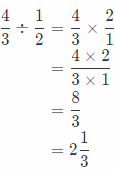We can see that result is mixed number.
The original fraction was greater than $$\frac{1}{2}$$

Question 20.
Communicate Mathematical Ideas The reciprocal of a fraction less than 1 is always a fraction greater than 1. Why is this?
For example, fraction $$\frac{1}{3}$$ is less than 1. Reciprocal of this fraction is $$\frac{3}{1}$$ = 3 and it is greater than 1.
In general, reciprocal of a fraction which is less than 1 will always be greater than 1 because nominator and the denominator switches and we get fraction which is greater than 1, always.
$$\frac{1}{3}$$ < 1 ⇒ $$\frac{3}{1}$$ = 3 > 1

Question 21.
Make a Prediction Susan divides the fraction $$\frac{5}{8}$$ by $$\frac{1}{16}$$. Her friend Robyn divides $$\frac{5}{8}$$ by $$\frac{1}{32}$$. Predict which person will get the greater quotient. Explain and check your prediction.
Robyn will get the greater quotient because he will actually multiply the same fraction as Susan, $$\frac{5}{8}$$, by greater fraction, it is reciprocal of $$\frac{1}{32}$$ and reciprocal of $$\frac{1}{32}$$ is 32, while Susan will multiply the same fraction but smaller number, by reciprocal of $$\frac{1}{16}$$ it is 16, and 16 is less than 32